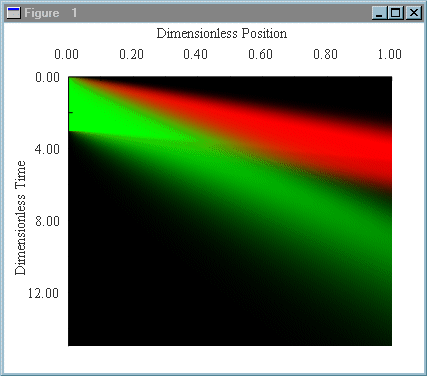Chromulator-IEX

# How to Get the Software?

Chromulator-IEX has been used by top biotech companies such as Amgen and Genentech. Both academic and commercial buyers need to pay a license fee (\$3k for commercial buyers). Academic discount is available. Buyer pays our invoice with a check payable to "Ohio University Foundation." Please e-mail Prof. Tingyue Gu (gu@ohio.edu) for details.

# Overview

Chromulator-IEX solves the general rate model for ion-exchange chromatography, and provides several methods of visualizing the solution.  The solution data can be exported to a Matlab® m-file or a text file.  It can be used to model a variety of IE applications including water treatment and protein separations.  The data can be visualized as effluent histories (chromatograms), position-time plots, and animations displaying the changing column profiles with time.

# Model

## Mass transfer

The flow through the column is assumed to be axial, with perfect radial mixing in the column.  Axial dispersion is described by the dimensionless Peclet number (Pe).  Mass transfer from the bulk mobile phase to the particle surfaces is described by the Biot number (Bi).  Diffusion within the particle pores is accounted for by the eta number (lower-case eta).

## Equilibrium

The mass-action isotherm is used to describe the ion-exchange equilibrium.  An equilibrium constant is specified for each component except the first, with the first component being the reference species.  The equilibrium constant can also be specified so that its logarithm is a polynomial function of the pH.  In addition, the absolute charge (lower-case nu) for each component is specified.  This can be a constant or a polynomial function of the pH.  The steric factor (lower-case sigma) allows for the Steric Mass-Action (SMA) isotherm to be used.  When all of the steric factors are zero, the isotherm reduces to the mass-action equations.  The equations for the isotherm are: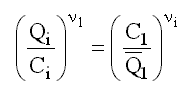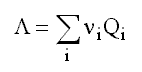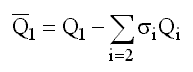In the above equations, Qi, is the adsorbed concentration of species 1, Ci is the its fluid-phase concentration, nui is the characteristic charge of species i, Lambda is the ion exchanger capacity in equivalents per unit particle skeleton, and sigmai is the steric factor for species i.  Q1 with an overbar is the concentration of species 1 available for exchange (i.e., not blocked by a large adsorbed molecule).

# Solution Procedure

The equations are solved numerically using a combination of finite elements, orthogonal collocation, and a stiff ordinary equation solver.  A Galerkin formulation with uniform quadratic finite elements is used to discretize the bulk mobile phase equations.  Orthogonal collocation is used to discretize the particle phase equations.  This results in a set of Ns(2Ne+1)(2Nc+1) ordinary differential equations, where Ns is the number of species, Ne is the number of finite elements, and Nc is the number of interior collocation points.  The VODE solver provided by Lawrence Livermore National Laboratory is used to solve this set of equations.  The local error tolerance for the ODE solver can be specified.  In practice, accurate solutions can usually be obtained with 15-30 elements and 1-3 internal collocation points, and an ODE error tolerance of 10-5.

#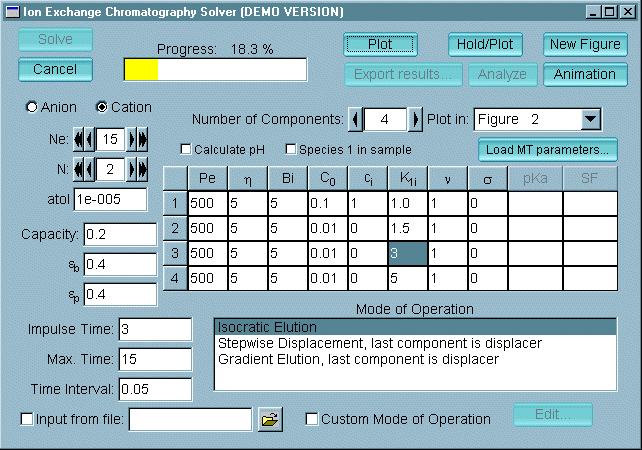User Interface

The user interface allows the system parameters, the parameters for each of the components, and the parameters for the numerical solution procedure to be entered.

The main window provides a graphical interface in which all of the simulation parameters can be specified.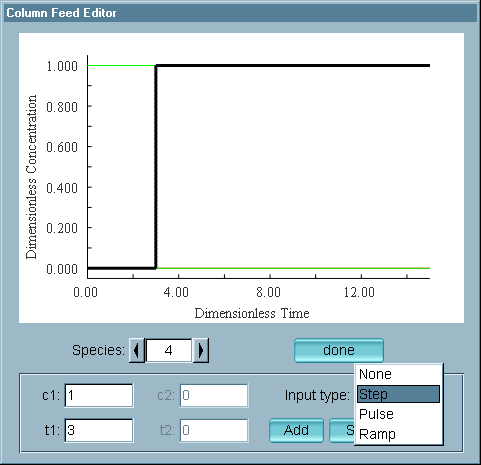The column feed can be specified by using one of the built-in modes of operation (isocratic elution, step displacement, or gradient elution), as a values interpolated from a

text file, or using the feed editor window to specify a sum of step, pulse, and ramp functions.

# Output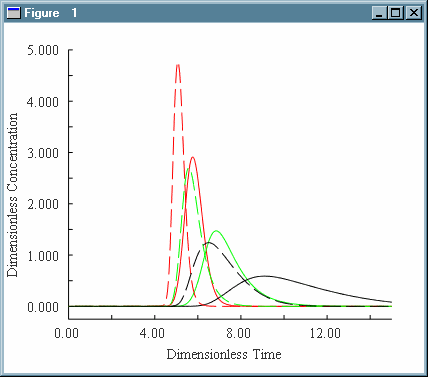A plot of the effluent history for a ternary separation is shown with the displacer salt displayed in blue.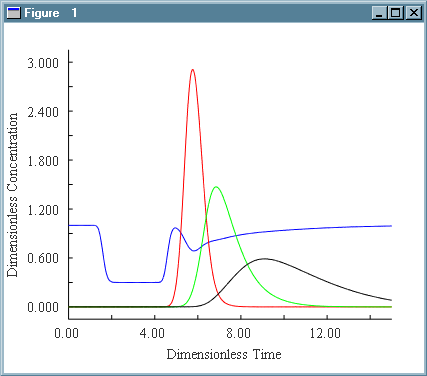Two ternary separations are displayed, with one data solution plotted with dotted lines.  The displacing salt is not shown.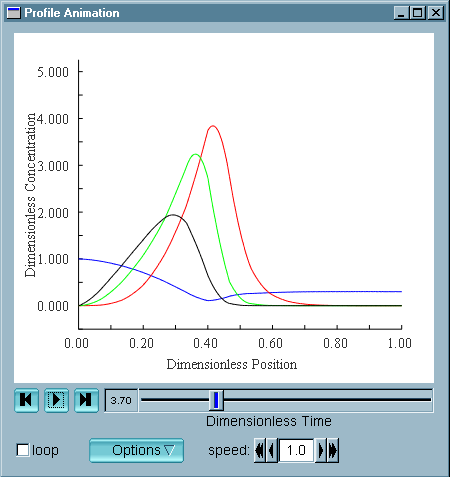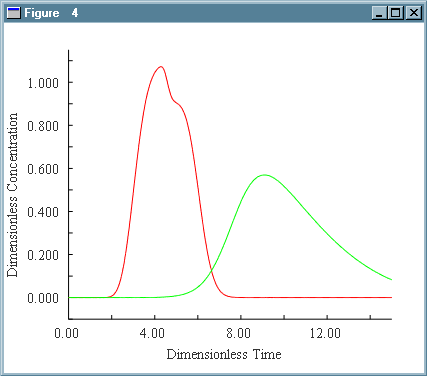Above left is the effluent history of a binary separation.  To the right is the position-time plot for this same separation.

The animation window allows the column profiles to be examined at specific times.  The changing column profiles can be viewed like a movie.